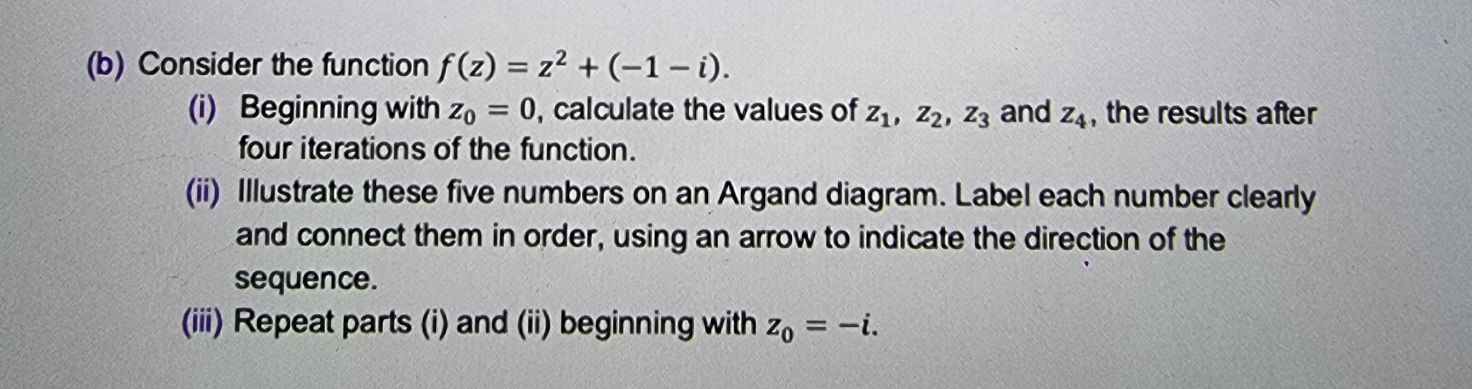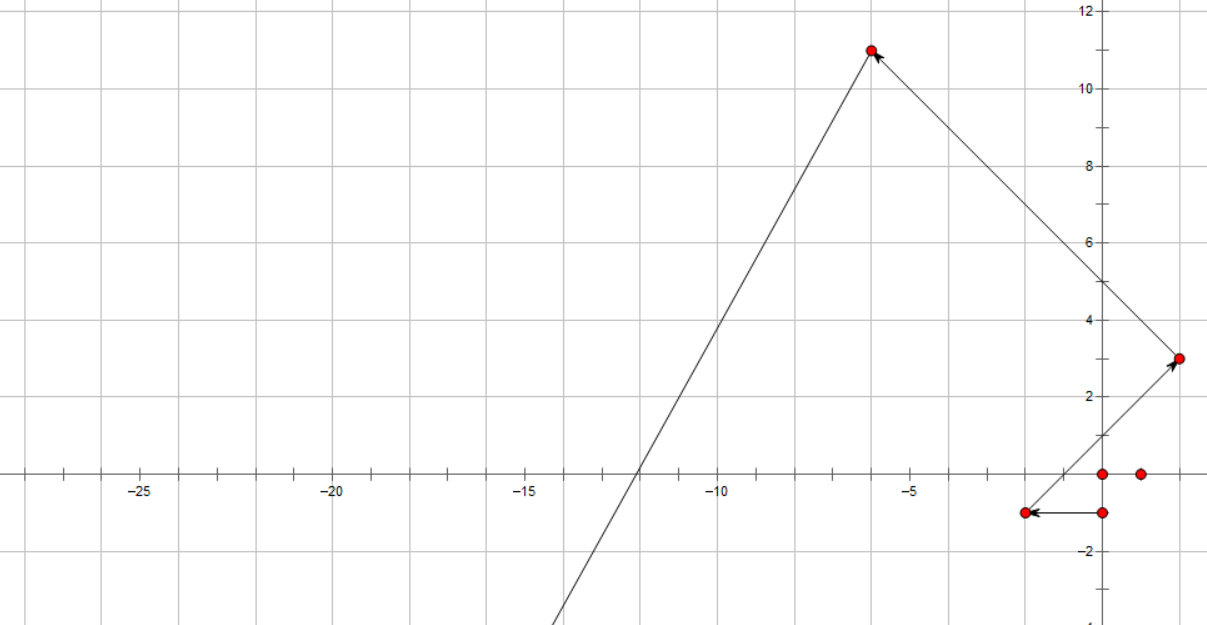### Still have math questions?

Algebra
Question(b) Consider the function $$f ( z ) = z ^ { 2 } + ( - 1 - i )$$ .

(i) Beginning with $$z _ { 0 } = 0$$ , calculate the values of $$z _ { 1 } , z _ { 2 } , z _ { 3 }$$ and $$z _ { 4 }$$ , the results after four iterations of the function.

(ii) Illustrate these five numbers on an Argand diagram. Label each number clearly and connect them in order, using an arrow to indicate the direction of the sequence.

(iii) Repeat parts (i) and (ii) beginning with $$z _ { 0 } = - i$$ .

$$z_1= -2- i\\z_2= 2+ 3i\\z_3= -6+ 11i\\z_4= -86- 133i$$